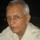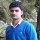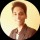Tough Puzzles & Brain Teasers
Puzzles on logic and mathematics that will make you scratch your head.
76 Members
Join this group to post and comment.28 Jan 2019

# 1 + 4 = 5, 2 + 5 = 12, 3 + 6 = 21 .... Solve this!

Here's an easy one for those who can quickly recognize the pattern -

1 + 4 = 5

2 + 5 = 12

3 + 6 = 21

5 + 8 = ?

PS: Please post your answer, but do not spoil the fun for others. 😬1mo ago

I shall have to

FortifyVijay Khatri
Computer Science
1mo ago

# 1 + 4 = 5, 2 + 5 = 12, 3 + 6 = 21 .... Solve this!Here's an easy one for those who can quickly recognize the pattern -1 + 4 = 52 + 5 = 123 + 6 = 215 + 8 = ?Answer:Very Simple the process going on 1+4=51*4+1=52 + 5 = 122*5+2=123 + 6 = 213*6+3=215 + 8 = ?5*8+5=45So 45 is the correct answer1mo ago

1+4=5

(5)+2+5=12

(12)+3+6=21

(21)+5+8=34Kunal Gokhe
Mechanical
1mo ago

5+8x5=45